summary of site-wide JavaScript functionalityUnited States-English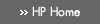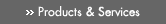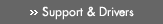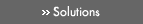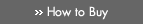» Contact HPSearch:More optionsManualTechnical documentation - EnglishAll of HP USHP-UX Reference > A# atan2(3M)

HP-UX 11i Version 3: February 2007»

## Technical documentation» Feedback## » Index## NAME

atan2(), atan2f(), atan2l(), atan2w(), atan2q() — arctangent and quadrant functions

## SYNOPSIS

#include <math.h>

double atan2(double y, double x);

float atan2f(float y, float x);

### HP Integrity Server Only

long double atan2l(long double y, long double x);

extended atan2w(extended y, extended x);

## DESCRIPTION

atan2() returns the arctangent of y/x, in the range -π to π, using the signs of both arguments to determine the quadrant of the return value.

atan2f() is a float version of atan2(); it takes float arguments and returns a float result.

### Integrity Server Only

atan2l() is a long double version of atan2(); it takes long double arguments and returns a long double result.

atan2w() is an extended version of atan2(); it takes extended arguments and returns an extended result.

atan2q() is equivalent to atan2l() on HP-UX systems.

## USAGE

To use these functions, compile either with the default -Ae option or with the -Aa and the -D_HPUX_SOURCE options.

To use (for Integrity servers) atan2w() or atan2q(), compile also with the -fpwidetypes option.

To use any of these functions, make sure your program includes <math.h>, and link in the math library by specifying -lm on the compiler or linker command line.

For more information, see the HP-UX floating-point guide for HP Integrity servers at the following site: http://www.hp.com/go/fp.

### PA-RISC Only

Millicode versions of the atan2() function are available. Millicode versions of math library functions are usually faster than their counterparts in the standard library. To use these versions, compile your program with the +Olibcalls or the +Oaggressive optimization option.

For special cases, the millicode versions return the same values as their standard library counterparts (see the RETURN VALUE section).

## RETURN VALUE

If y is ±0 and x is -0 atan2() returns ±π respectively.

If y is ±0 and x is +0 atan2() returns ±0 respectively.

If y is ±0 and x is less than zero, atan2() returns returns ±π respectively.

If y is ±0 and x is greater than zero, atan2() returns returns ±0 respectively.

If y is less than zero and x is zero, atan2() returns -π/2.

If y is greater than zero and x is zero, atan2() returns π/2.

If y is greater than zero and x is -INFINITY, atan2() returns Pi.

If y is less than zero and x is -INFINITY, atan2() returns -π.

If y is greater than zero and x is INFINITY, atan2() returns +0.

If y is less than zero and x is INFINITY, atan2() returns -0.

If y is ±INFINITY and x is finite, atan2() returns ±π/2 respectively.

If y is ±INFINITY and x is -INFINITY, atan2() returns ±3π/4 respectively.

If y is ±INFINITY and x is +INFINITY, atan2() returns ±π/4 respectively.

If x or y is NaN, atan2() returns NaN.

Whether atan2() raises the inexact exception is unspecified.

## ERRORS

No errors are defined.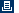Printable versionPrivacy statement Using this site means you accept its terms Feedback to webmaster© 1983-2007 Hewlett-Packard Development Company, L.P.